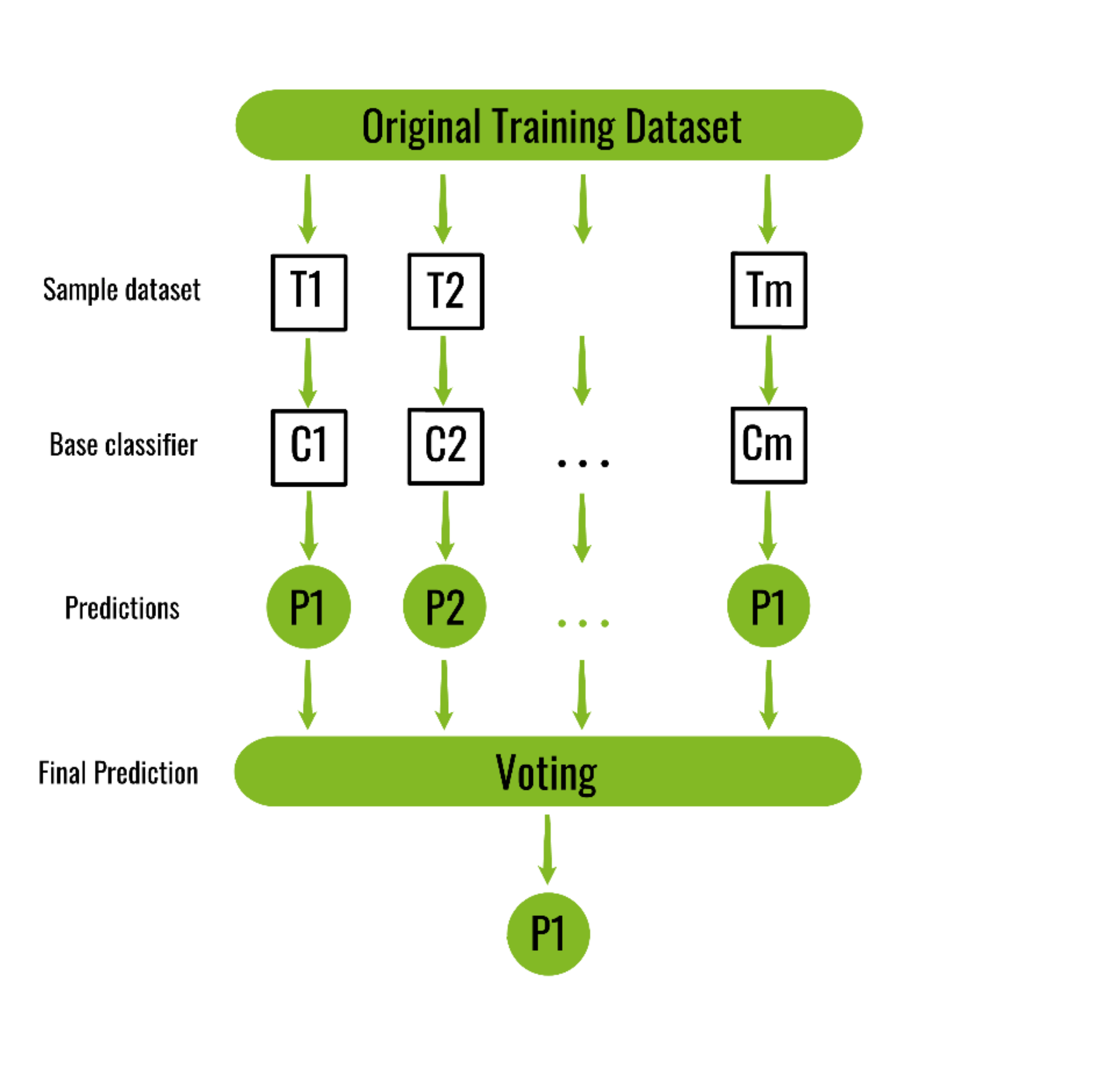# ML | Bagging classifier

A Bagging classifier is an ensemble meta-estimator that fits base classifiers each on random subsets of the original dataset and then aggregate their individual predictions (either by voting or by averaging) to form a final prediction. Such a meta-estimator can typically be used as a way to reduce the variance of a black-box estimator (e.g., a decision tree), by introducing randomization into its construction procedure and then making an ensemble out of it.
Each base classifier is trained in parallel with a training set which is generated by randomly drawing, with replacement, N examples(or data) from the original training dataset – where N is the size of the original training set. Training set for each of the base classifiers is independent of each other. Many of the original data may be repeated in the resulting training set while others may be left out.

Bagging reduces overfitting (variance) by averaging or voting, however, this leads to an increase in bias, which is compensated by the reduction in variance though.

How Bagging works on training dataset ?
How bagging works on an imaginary training dataset is shown below. Since Bagging resamples the original training dataset with replacement, some instance(or data) may be present multiple times while others are left out.

Original training dataset: 1, 2, 3, 4, 5, 6, 7, 8, 9, 10

Resampled training set 1: 2, 3, 3, 5, 6, 1, 8, 10, 9, 1
Resampled training set 2: 1, 1, 5, 6, 3, 8, 9, 10, 2, 7
Resampled training set 3: 1, 5, 8, 9, 2, 10, 9, 7, 5, 4

Algorithm for the Bagging classifier:

```Classifier generation:

Let N be the size of the training set.
for each of t iterations:
sample N instances with replacement from the original training set.
apply the learning algorithm to the sample.
store the resulting classifier.

Classification:
for each of the t classifiers:
predict class of instance using classifier.
return class that was predicted most often.
```Below is the Python implementation of the above algorithm:

 `from` `sklearn ``import` `model_selection ` `from` `sklearn.ensemble ``import` `BaggingClassifier ` `from` `sklearn.tree ``import` `DecisionTreeClassifier ` `import` `pandas as pd ` ` `  `# load the data ` `url ``=` `"/home/debomit/Downloads/wine_data.xlsx"` `dataframe ``=` `pd.read_excel(url) ` `arr ``=` `dataframe.values ` `X ``=` `arr[:, ``1``:``14``] ` `Y ``=` `arr[:, ``0``] ` ` `  `seed ``=` `8` `kfold ``=` `model_selection.KFold(n_splits ``=` `3``, ` `                       ``random_state ``=` `seed) ` ` `  `# initialize the base classifier ` `base_cls ``=` `DecisionTreeClassifier() ` ` `  `# no. of base classifier ` `num_trees ``=` `500` ` `  `# bagging classifier ` `model ``=` `BaggingClassifier(base_estimator ``=` `base_cls, ` `                          ``n_estimators ``=` `num_trees, ` `                          ``random_state ``=` `seed) ` ` `  `results ``=` `model_selection.cross_val_score(model, X, Y, cv ``=` `kfold) ` `print``(``"accuracy :"``) ` `print``(results.mean()) `

Output:

```accuracy :
0.8372093023255814
```

My Personal Notes arrow_drop_upCheck out this Author's contributed articles.

If you like GeeksforGeeks and would like to contribute, you can also write an article using contribute.geeksforgeeks.org or mail your article to contribute@geeksforgeeks.org. See your article appearing on the GeeksforGeeks main page and help other Geeks.

Please Improve this article if you find anything incorrect by clicking on the "Improve Article" button below.

Article Tags :
Practice Tags :

1

Please write to us at contribute@geeksforgeeks.org to report any issue with the above content.